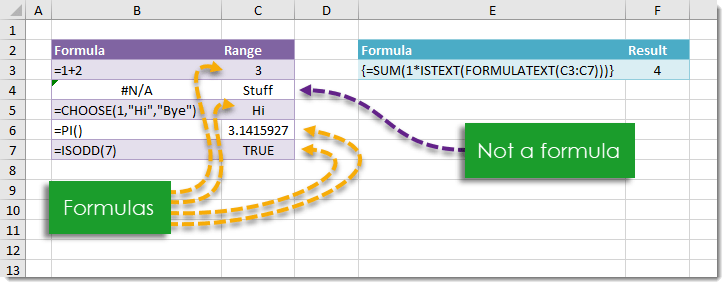## Example

{=SUM(1*ISTEXT(FORMULATEXT(C3:C7)))}

## Generic Formula

{=SUM(1*ISTEXT(FORMULATEXT(Range)))}

Note: This is an array formula. Do not type out the {} brackets. Hold Ctrl + Shift then press Enter while in Edit Mode to create an array formula.

Range – This is the range in which you want to count the number of cells containing a formula.

## What It Does

This formula will return a count of the number of cells in a given range which contain a formula.

## How It Works

We use the FORMULATEXT(Range) to create an array which contains a text string of each formula from the Range. When a cell in the Range does not contain a formula FORMULATEXT will return an #N/A error value.

We use 1*ISTEXT to return a 1 for any text strings and 0 for #N/A errors. We then SUM the result up and this is our count of cells containing a formula.

In our example FORMULATEXT(Range) results in the following array.

{“=1+2″;#N/A;”=CHOOSE(1,”Hi”,”Bye”)”;”=PI()”;”=ISODD(7)”}

ISTEXT({“=1+2″;#N/A;”=CHOOSE(1,”Hi”,”Bye”)”;”=PI()”;”=ISODD(7)”}) will return an array with TRUE values when there is a text string and FALSE values when there is an #N/A error value.

In our example this results in {TRUE;FALSE;TRUE;TRUE;TRUE}.

SUM(1*{TRUE;FALSE;TRUE;TRUE;TRUE}) becomes SUM({1;0;1;1;1}) because 1*TRUE is 1 and 1*FALSE is 0. SUM({1;0;1;1;1}) = 4 is the count of cells containing a formula in our range.